# Quiz 2: Developing Your Financial Statements and Plans

Business

a) Rent Paid is listed as an expense. The total amount of rent paid for the year would be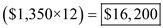unless he has some amount as outstanding. b) The diamond ear rings will be assets for the company. And the amount of \$900 which is not yet paid will be treated as a current liability for the company. c) The amount of \$3,500 , received from his parents will be treated as income. And the repayment of the same will be shown as liability on balance sheet. d) Assuming he made 12 payments during the year, therefore total loan payments of \$2,700 will be treated as expense. Of the 20 remaining payments, only about half are for principal. Therefore, on the balance sheet he should show the unpaid principal of about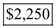as liability's which is calculated as follows:e) Here, \$3,800 will be shown as an expense on the income and expense account. But the tax refund of \$550 will not show as an income because it has not yet received. f) The amount of \$2,300 will be shown as an expense on income and expenditure account. And also as an investment in the balance sheet.

a) Mr. S is correct in suggesting that, only the amount of \$3,917 is shown as income. But if they choose to show the tax expense then Mrs. E would be correct showing an amount of \$5,000 as income including the expense of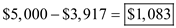b) By having an allowance for "fun money" Mrs. E has specifically set aside a certain portion of their income for a little self-satisfaction. This will serve two basic purposes: • It will give little financial independence. • It may allow Mr. S for some impulse buying.

a) To obtain the value of the investment worth \$45,000 today, which pays at a7 percent interest rate by the end of 25years, calculate its future value using the following equation which is as follows: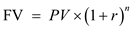…… (1) Substitute \$25,000 for ' PV ', 0.07 percent for ' r ', and 25 years for ' n 'Hence, the future value of \$45,000 will be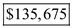b) The cost of home after 10years, which costs \$275,000 today, if the price goes up by 5percent yearly, calculate its future value using the equation 1 as follows: Substitute \$275,000 for ' PV ', 0.05 percent for ' r ', 10 years for ' n 'Hence, the future value of \$375,000 will be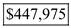c) If \$75,000 is invested at 4 Percent interest rate, then after 15years the value of the investment can be calculated using the equation 1 as follows: Substitute \$75,000 for ' PV ', 0.04 percent for ' r ', and 15 years for ' n 'Therefore, the future value of the investment is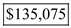Now, subtract the cost form the future value as follows: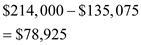Therefore, from the above calculation it can be concluded that, the individual is in deficit of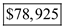Now, calculate present value for the cost to find out the amount an individual has to invest today to meet the cost of \$214,000, using the following equation as follows: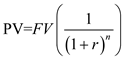…… (2) Substitute \$214,000 for ' FV ', 0.04 percent for ' r ', and 15 years for ' n '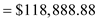Therefore, from the above calculations it can be concluded that, an individual has to invest an amount of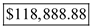, in order to meet the cost of \$214,000 at 4 percent interest rate for 15 years. d) Calculate Future value of annuity for the given information using the following equation, which is as follows: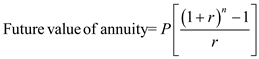…… (3) Substitute \$1,000,000 for ' P ', 0.04 percent for ' r ', and 35 years for ' n '\Therefore, form the above calculations it can be concluded that, an individual have to save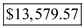at the end of each year at a rate of 4 percent for the next 35years in order to retire with \$1million.